# ttk基本知识 - tkinter中文手册

ttk组件在外观样式上是跟系统相统一的主题，在外观上好看多了，但要注意，ttk在外观样式的设置上，跟tkinter模块的组件有些不一样，它可以靠ttk的style类来实现，作为后续的ttk模块对tkinter的兼容性并不好，比如，以前我们设置背景颜色bg，前景颜色fg，就不可以用了。

```import tkinter as tk
import tkinter.ttk as ttk

root=tk.Tk()

fr1=tk.LabelFrame(root,text='tk.LableFrame') # 不设置边线宽，无法显示
fr2=ttk.LabelFrame(root,text='ttk.LableFrame') # 不设置边线宽，无法显示

but1=tk.Button(fr1,text="按钮1")

but2=ttk.Button(fr2,text="按钮2")

la1=tk.Label(fr1,text='标签1',bg='lightblue')

la2=ttk.Label(fr2,text='标签2',background='lightblue')

var1=tk.IntVar()

var2=tk.IntVar()
ch1=tk.Checkbutton(fr1,text="多选按钮1",variable=var1)

var3=tk.IntVar()
ch2=ttk.Checkbutton(fr2,text="多选按钮1",variable=var3)

sca1=tk.Scale(fr1,from_=0,to=255,orient=tk.HORIZONTAL)

sca2=ttk.Scale(fr2,from_=0,to=255,orient=tk.HORIZONTAL)

root.mainloop()```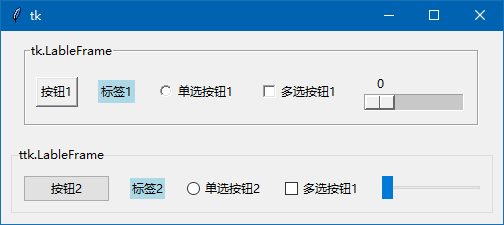`la2=ttk.Label(fr2,text='标签2',background='lightblue')`

```print(la1.keys())
print('----------------------')
print(la2.keys())```

['activebackground', 'activeforeground', 'anchor', 'background', 'bd', 'bg', 'bitmap', 'borderwidth', 'compound', 'cursor', 'disabledforeground', 'fg', 'font', 'foreground', 'height', 'highlightbackground', 'highlightcolor', 'highlightthickness', 'image', 'justify', 'padx', 'pady', 'relief', 'state', 'takefocus', 'text', 'textvariable', 'underline', 'width', 'wraplength']

----------------------

['background', 'foreground', 'font', 'borderwidth', 'relief', 'anchor', 'justify', 'wraplength', 'takefocus', 'text', 'textvariable', 'underline', 'width', 'image', 'compound', 'padding', 'state', 'cursor', 'style', 'class']

style()方法，设置

```from tkinter import *
from tkinter.ttk import *

root=Tk()

but1=Button(root,text="wb98.com",background='blue',foreground='red',font=('黑体',12,'bold'))
but1.pack()

root.mainloop()```

Style对象创建方法： 对象名=Style()

style1=Style()

s2=Style()

wb98=Style()

Style对象设置方法：对象实例.configure('ttk样式名', 样式参数......)

style1.configure('TButton', background='blue',foreground='red')

s2.configure('my.TButton', background='blue',foreground='red')

wb98.configure('.', background='blue',foreground='red')

例如：Style对象名. configure('.', background='blue',foreground='red')

设置成功后，窗体上的所有组件：按钮，标签，单选按钮……等都被设置成蓝色背景色，红色前景色。

 组件类 ttk样式名 Button TButton Checkbutton TCheckbutton Radiobutton TRadiobutton Entry TEntry Combobox TCombobox Frame TFrame Label TLabel LabelFrame TLabelFrame Notebook TNtebook Menubutton TMenubutton PanedWindow TPandwindow   ( 不是TpandWindow ) Progressbar Horizontal.TProgressbar   Vertical.TProgressbar Scale Horizontal.TScale      Vertical.TScale Scrollbar Horizontal.TScrollbar    Vertical.TScrollbar Sizegrip TSizegrip Separator TSeparator Treeview Treeview    ( 不是 TTreeview  )

我的一个标签取样式名：wb86.TLabel

```from tkinter import *
from tkinter.ttk import *

root=Tk()

but1=Button(root,text="我是按钮1")

but2=Button(root,text="我是按钮2")

la1=Label(root,text='我是标签')

root.mainloop()```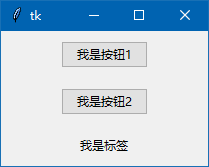```from tkinter import *
from tkinter.ttk import *

root=Tk()

style1 = Style() # 创建Style对象，便于下面设置样式
style1.configure('.',background='blue',foreground='red',font=('黑体',12))
#设置背景颜色为蓝色，前景颜色为红色,'.'为所有组件的样式名

but1=Button(root,text="我是按钮1")

but2=Button(root,text="我是按钮2")

la1=Label(root,text='我是标签')

root.mainloop()```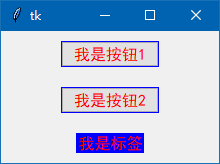```from tkinter import *
from tkinter.ttk import *

root=Tk()

style1 = Style() # 创建Style对象，便于下面设置样式
style1.configure('TButton',background='blue',foreground='red',font=('黑体',12))
#设置背景颜色为蓝色，前景颜色为红色,'TButton'为所有按钮组件的样式名

but1=Button(root,text="我是按钮1")

but2=Button(root,text="我是按钮2")

la1=Label(root,text='我是标签')

root.mainloop()```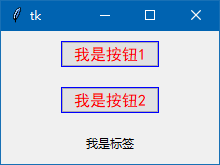```from tkinter import *
from tkinter.ttk import *

root=Tk()

style1 = Style() # 创建Style对象，便于下面设置样式
style1.configure('my.TButton',background='blue',foreground='red',font=('黑体',12))
#设置背景颜色为蓝色，前景颜色为红色,'my.TButton'为部分按钮组件的样式名

but1=Button(root,text="我是按钮1",style='my.TButton')

but2=Button(root,text="我是按钮2")

la1=Label(root,text='我是标签')

root.mainloop()```测试成功，2个按钮中，只有按钮1样式有变化，另一个按钮是默认的样式，标签也是默认的样式。

```from tkinter import *
from tkinter.ttk import *

root=Tk()

style1 = Style() # 创建Style对象，便于下面设置样式
print(style1.theme_names()) # 输出本机ttk所有主题风格
print(style1.theme_use()) # 输出现在采用的那种主题

root.mainloop()```

('winnative', 'clam', 'alt', 'default', 'classic', 'vista', 'xpnative')

vista

```from tkinter import *
from tkinter.ttk import *

root=Tk()  # 源码来自wb98.com

root.columnconfigure(0,weight=1,minsize=150) # 0列 限制最小宽度为150像素
root.columnconfigure(1,weight=1,minsize=150) # 1列 限制最小宽度为150像素

fr1=Frame(root) # fr1是作为窗体的底色而创建的
fr1.place(x=0,y=0,relwidth=1,relheight=1) # 参数保证fr1跟窗体一样大小

style1 = Style() # 创建Style对象，便于下面设置样式

tuple_op=style1.theme_names() # 返回值是本机ttk所有主题风格的元组
var=StringVar()

def changejob(cs): # 参数正好就是选择项的文本
style1.theme_use(cs)

op1.grid()
var.set(style1.theme_use()) # 组件默认选项设为目前的主题

lfr1=LabelFrame(root,text='单选') # 装入3个单选按钮

var1=IntVar()
var1.set(1)

lfr2=LabelFrame(root,text='多选') # 装入3个多选按钮
var2,var3,var4=IntVar(),IntVar(),IntVar()
ch1=Checkbutton(lfr2,text="足球",variable=var2).pack()
ch2=Checkbutton(lfr2,text="田径",variable=var3).pack()
ch3=Checkbutton(lfr2,text="游泳",variable=var4).pack()
var3.set(True)
var4.set(True)

fr1=Frame(root) # 框架装入2个按钮

but1=Button(fr1,text=" 退 出 ",command=root.destroy)
but2=Button(fr1,text=" 确 定 ")
but2.pack(side=RIGHT)

root.mainloop()```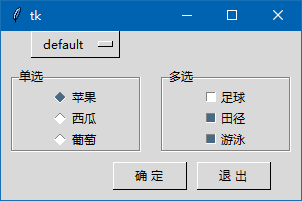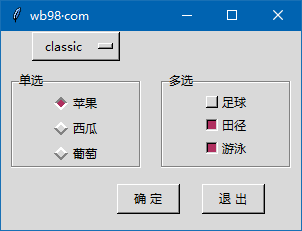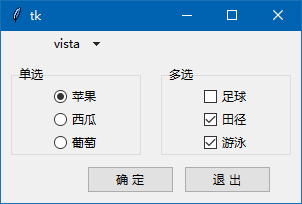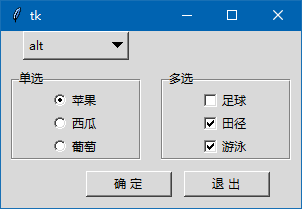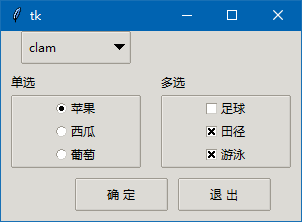<< 上一篇 下一篇 >>